Publicité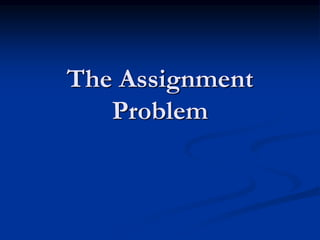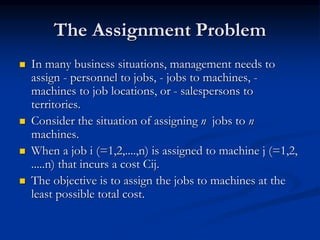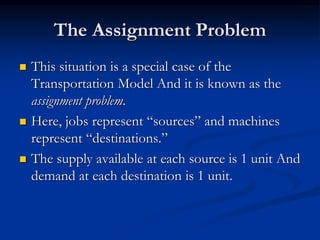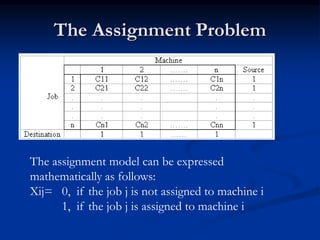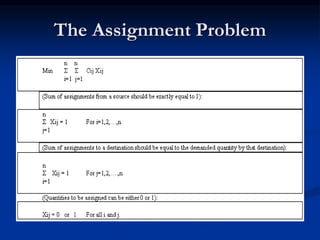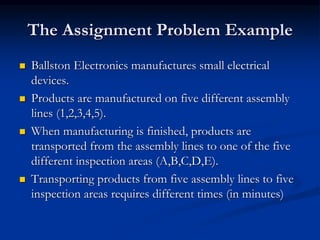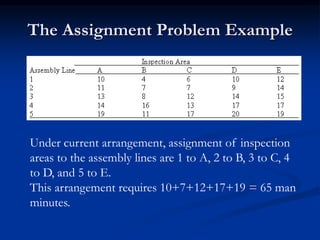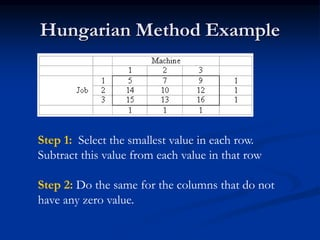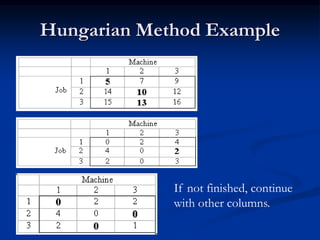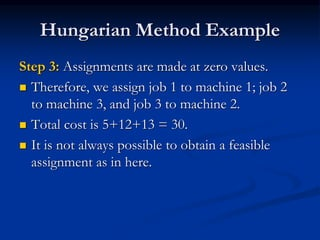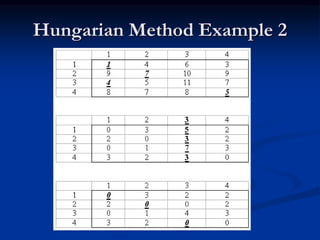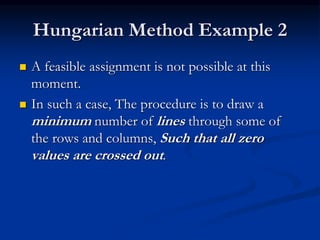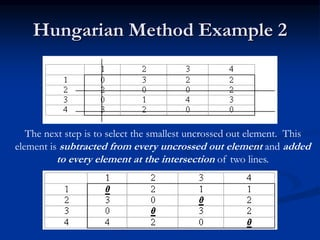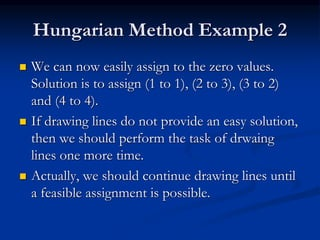1 sur 14
Publicité

### E1605_ASSIGNMENT_PROBLEM.ppt

1. The Assignment Problem
2. The Assignment Problem  In many business situations, management needs to assign - personnel to jobs, - jobs to machines, - machines to job locations, or - salespersons to territories.  Consider the situation of assigning n jobs to n machines.  When a job i (=1,2,....,n) is assigned to machine j (=1,2, .....n) that incurs a cost Cij.  The objective is to assign the jobs to machines at the least possible total cost.
3. The Assignment Problem  This situation is a special case of the Transportation Model And it is known as the assignment problem.  Here, jobs represent “sources” and machines represent “destinations.”  The supply available at each source is 1 unit And demand at each destination is 1 unit.
4. The Assignment Problem The assignment model can be expressed mathematically as follows: Xij= 0, if the job j is not assigned to machine i 1, if the job j is assigned to machine i
5. The Assignment Problem
6. The Assignment Problem Example  Ballston Electronics manufactures small electrical devices.  Products are manufactured on five different assembly lines (1,2,3,4,5).  When manufacturing is finished, products are transported from the assembly lines to one of the five different inspection areas (A,B,C,D,E).  Transporting products from five assembly lines to five inspection areas requires different times (in minutes)
7. The Assignment Problem Example Under current arrangement, assignment of inspection areas to the assembly lines are 1 to A, 2 to B, 3 to C, 4 to D, and 5 to E. This arrangement requires 10+7+12+17+19 = 65 man minutes.
8. Hungarian Method Example Step 1: Select the smallest value in each row. Subtract this value from each value in that row Step 2: Do the same for the columns that do not have any zero value.
9. Hungarian Method Example If not finished, continue with other columns.
10. Hungarian Method Example Step 3: Assignments are made at zero values.  Therefore, we assign job 1 to machine 1; job 2 to machine 3, and job 3 to machine 2.  Total cost is 5+12+13 = 30.  It is not always possible to obtain a feasible assignment as in here.
11. Hungarian Method Example 2
12. Hungarian Method Example 2  A feasible assignment is not possible at this moment.  In such a case, The procedure is to draw a minimum number of lines through some of the rows and columns, Such that all zero values are crossed out.
13. Hungarian Method Example 2 The next step is to select the smallest uncrossed out element. This element is subtracted from every uncrossed out element and added to every element at the intersection of two lines.
14. Hungarian Method Example 2  We can now easily assign to the zero values. Solution is to assign (1 to 1), (2 to 3), (3 to 2) and (4 to 4).  If drawing lines do not provide an easy solution, then we should perform the task of drwaing lines one more time.  Actually, we should continue drawing lines until a feasible assignment is possible.
Publicité